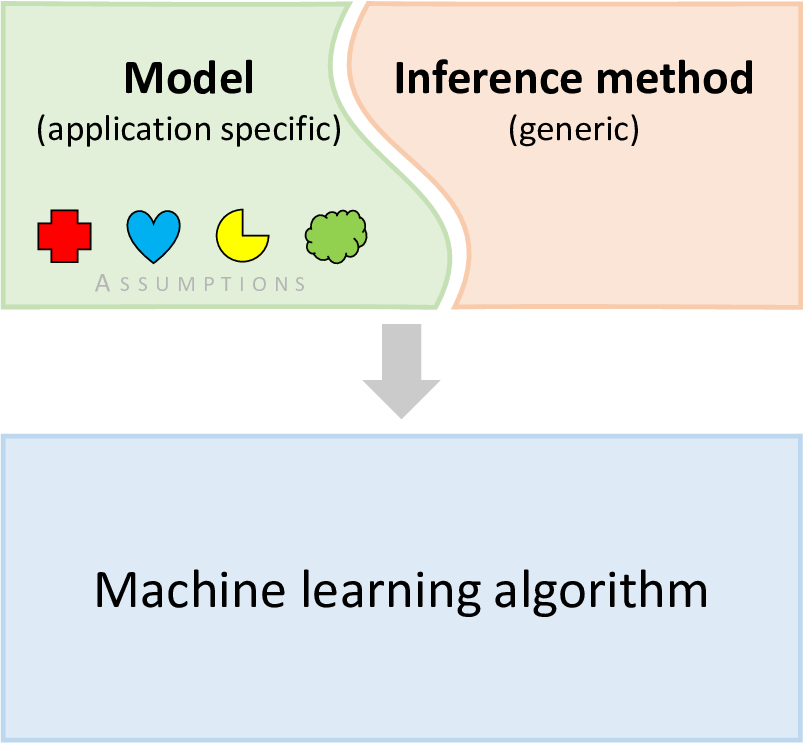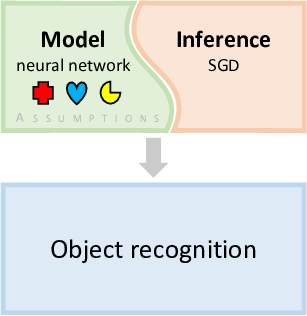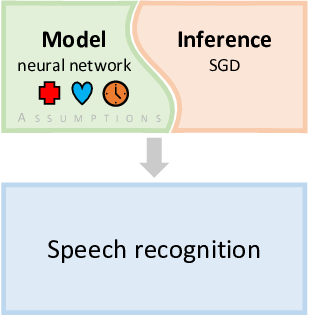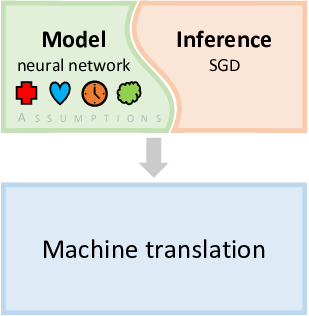# How can machine learning solve my problem?

As machine learning researchers at Microsoft, there’s a question that we get asked in some form almost every day:

“How can machine learning solve my problem?”

In this book we answer this question by example. We do not just list machine learning techniques and concepts – instead we describe a series of case studies, all the way through from problem statement to working solution. Machine learning concepts are explained as they arise in the context of solving each problem. The case studies we present are all real examples from within Microsoft, along with an initial case study which introduces some core concepts. We also look at real problems encountered during each case study, how they were detected, how they were diagnosed and how they were overcome. The aim is to explain not just what machine learning methods are, but also how to create, debug and evolve them to solve your problem.Machine learning can seem daunting to newcomers.

During the last few years, machine learning has moved to centre stage in the world of technology. Today, thousands of engineers and researchers are applying machine learning to an extraordinarily broad range of domains. However, making effective use of machine learning in practice can be daunting, especially for newcomers to the field. When someone is trying to solve a real-world problem using machine learning, they often encounter challenges:

• “I am overwhelmed by the choice of machine learning methods. There’s too much to learn!”
• “I don’t know which algorithm to use or why one would be better than another for my problem.”
• “My problem doesn’t seem to fit with any standard algorithm.”

In this book we apply an approach to machine learning which we call model-based machine learning. Model-based machine learning helps to address all of these challenges, and makes the process of creating effective machine learning solutions much more transparent.

### What is model-based machine learning?

Over the last five decades, researchers have created literally thousands of machine learning algorithms Each algorithm is a series of instructions which the computer follows to do a machine learning task, such as prediction. A machine learning engineer or scientist wanting to solve a problem must choose an algorithm to try, or otherwise attempt to invent a new one. In practice, their choice will be constrained by those algorithms they happen to be familiar with, or by the availability of specific software, and may not be the best choice for their problem.

By contrast the model-based approach seeks to create a bespoke solution tailored to each new application. Instead of having to transform a problem to fit some standard algorithm, model-based machine learning provides a custom algorithm to precisely fit the problem.

The core idea at the heart of model-based machine learning is that all the assumptions about the problem domain are made explicit in the form of a model. In fact, a model is just made up of this set of assumptions, expressed in a precise mathematical form. These assumptions include the number and types of variables in the problem domain, which variables affect each other, and what the effect of changing one variable is on another variable. For example, in the next chapter we build a model to help us solve a simple murder mystery. The assumptions of the model include the list of suspected culprits, the possible murder weapons, and the tendency for particular weapons to be preferred by different suspects. This model is then used to create a bespoke algorithm to solve the specific machine learning problem. Model-based machine learning can be applied to pretty much any problem, and its general-purpose approach means you don’t need to learn a huge number of machine learning algorithms and techniques.

So why do the assumptions of the model play such a key role? Well it turns out that machine learning cannot generate solutions purely from data alone. There are always assumptions built into any algorithm, although sometimes these assumptions are far from explicit. Different algorithms correspond to different sets of assumptions. In cases when the assumptions are unclear, the only way to decide which algorithm will give the best results is to try each in turn. This is time-consuming and inefficient, and it requires software implementations of all of the algorithms being compared. And if none of the algorithms tried gives good results, it is even harder to work out how to create a better algorithm.

#### Models versus algorithms

Let’s look more closely at the relationship between models and algorithms. We can think of a machine learning algorithm as a monolithic box which takes in data and produces results. The algorithm must necessarily make assumptions, since it is these assumptions that distinguish a particular algorithm from any other. However, given just the algorithm, those assumptions are implicit and opaque.

Now consider the model-based approach. The model comprises the set of assumptions we are making about the problem domain. To get from the model to a set of predictions we need to take the data and compute those variables whose values we wish to know. This computational process is called inference. There are several techniques available for doing inference, as we shall discuss during the course of this book. The combination of the model and the inference procedure together define a machine learning algorithm, as illustrated in Figure 0.1.Figure 0.1In the model-based view of machine learning, a custom algorithm is created by combining a model and an inference method. Here the coloured shapes within the model represent the assumptions comprising that specific model. Changes to the assumptions give rise to different machine learning algorithms, even when the inference method is kept fixed.

Although there are various choices for the inference method, by decoupling the model from the inference we are able to apply the same inference method to a wide variety of models. To illustrate this point, every single case study in this book will be solved using just one inference method.

Model-based machine learning can be used to do any perform machine learning task, such as classification (Chapter 4) or clustering (Chapter 6), whilst providing additional insight and control over how these tasks are performed. Solving these tasks using model-based machine learning provides a way to handle extensions to the task or to improve accuracy, by making changes to the model – we will look at an example of this in Chapter 4. Additionally, the assumptions you are making about the problem domain are laid out clearly in the model, so it is easier to work out why one model works better than another, to communicate to someone else what a model is doing, and to understand what’s happening when things go wrong. Using models also makes it easier to share other people’s solutions in order to adapt, extend, or combine them.

#### An example: deep learning

In recent years, deep learning has become the dominant approach to machine learning to such an extent that, to many people, deep learning is machine learning. What is less well known is that deep learning is an example of model-based machine learning, where the model being used is a neural network. Assumptions about the problem domain are encoded in the architecture of the neural network and in the choice of activation function for the neurons. No matter what neural network model is chosen, the same inference methods can be applied. For example, a neural network is usually trained using some kinds of stochastic gradient descent (SGD) method. Combining a particular neural network with SGD effectively gives a custom algorithm for training to solve a particular machine learning problem.(a)(b)(c)

Figure 0.2In deep learning, different neural network models encode different assumptions about the task they are used for, illustrated here as different icons within each model. For example, the architecture of a neural network for object recognition encodes the assumption that objects look similar no matter where in the image they appear. Although the neural network models are different for different tasks, the same inference methods can be applied. Here, stochastic gradient descent (SGD) can be applied to train any of these models. Since the models are similar and the inference methods are the same, deep learning can be rapidly applied to new problem domains.

Figure 0.2 illustrates how deep learning has made use of model-based machine learning. One of the first breakthroughs in deep learning was when deep neural networks were used for object recognition in images [Krizhevsky et al., 2012]. The particular architecture of neural network chosen for this task encoded assumptions about the nature of objects in images – for example, that objects look similar no matter where in the image they appear. Combined with a suitable inference method, this gave a custom algorithm for object recognition which achieved unprecedented accuracy. For speech recognition this assumption does not make sense and so different architectures were used which made more appropriate assumptions – for example, that a particular word may be spoken quickly or slowly. However, other assumptions encoded in the form of the neural network were retained, since they are broadly applicable to many problem domains. The ability to retain many aspects of the neural network while making targeted changes has allowed deep learning to be applied to many different application areas relatively quickly , including machine translation [Sutskever et al., 2014]. Arguably, it is this ability, building on its model-based foundations, that has enabled the deep learning revolution.

#### Tools for model-based machine learning

The decomposition of an algorithm into a model and a separate inference method has another powerful consequence. It becomes possible to create a software framework which will generate the machine learning algorithm automatically, given only the definition of the model and a choice of inference method. This allows the applications developer to focus on the creation of the model, which is domain-specific, and frees them from needing to be an expert on the inner workings of the inference procedure.

For more than fifteen years we have been working on such a software framework at Microsoft Research, called Infer.NET [Minka et al., 2014]. Because a model consists simply of a set of assumptions it can be expressed in very compact code, which is relatively easy to understand and modify. The corresponding code for the algorithm, which is generally much more complex, is then produced automatically. All of the models in this book were created using Infer.NET, and the corresponding model source code is available online. However, these solutions could equally be implemented by hand or by using an alternative model-based framework – they are not specific to Infer.NET. Examples of alternative software frameworks that implement the model-based machine learning philosophy include BUGS [Lunn et al., 2000] and Stan [Stan Development Team, 2014].

As well as these general-purpose software frameworks, there has been enormous effort put in developing software specifically for neural network models, such as Tensorflow [Abadi et al., 2016] and PyTorch [Paszke et al., 2019]. Such frameworks embody the model-based machine learning approach by allowing the neural network to be described through a model description, such as in an ONNX file [Bai et al., 2019]. In this way, a custom neural network model can be trained or applied automatically, by any of the range of tools that support the ONNX format. This portability and ease-of-use are consequences of the model-based approach to machine learning.

### Who is this book for?

This book is unusual for a machine learning text book in that we do not review dozens of different algorithms. Instead we introduce all of the key ideas through case studies involving real-world applications. Case studies play a central role because it is only in the context of applications that it makes sense to discuss modelling assumptions. Each chapter introduces one case study which is drawn from a real-world application that has been solved using a model-based approach. In addition, a first tutorial chapter explores a fictional problem involving a murder mystery.Only a few building blocks are needed to construct an infinite variety of models.

Each chapter also serves to introduce a variety of machine learning concepts, not as abstract ideas, but as concrete techniques motivated by the needs of the application. You can think of these concepts as the building blocks for constructing models. Although you will need to invest some time to understand these concepts fully, you will soon discover that a huge variety of models can be constructed from a relatively small number of building blocks. By working through the case studies in this book you will learn how to use these components, and will hopefully gain a sufficient appreciation of the power and flexibility of model-based approach to allow you to solve your machine learning problem.

Inference deep-dive

This book is intended for any technical person who wants to use machine learning to solve a real-world problem – the focus of the book is on designing models to solve problems. However, some readers will also want to understand the mathematical details of how models are turned into inference algorithms. We have separated these parts of the book, which require more advanced mathematics, into inference deep-dive sections, which will be marked with panels like this one.

Deep-dive sections are optional – you can read the book without them. If you are planning on using a software framework like Infer.NET or just want to focus on modelling, you can skip these sections.

### How to read this book

Each case study in this book describes a journey from problem statement to solution. You probably do not want to follow this journey in a single sitting. To help with this, each case study is split into sections – we recommend reading a section at a time and pausing to digest what you have learned at the end of each section. To help with this, the machine learning concepts introduced in a section will be highlighted like this and will be reviewed at the end of each section (as you can see below). We aim to provide enough details of each concept to allow the case studies to be understood, along with links to external sources, such as Bishop , where you can get more details if you are interested in a particular topic.

Now, on to the first case study!

model-based machine learningAn approach to machine learning where all the assumptions about the problem domain are made explicit in the form of a model. This model is then used to create a model-specific to learn or reason about the domain. The algorithm creation part of this process can be automated.

algorithmA series of instructions used to solve a problem or perform a computation. Usually an algorithm is applied to some input data to produce some output.

modelA set of assumptions about a problem domain, expressed in a precise mathematical form, that is used to create a machine learning solution.

inferenceThe process of using a machine learning model to perform a task given some data. For example, inference may be applied to a model to make predictions or to learn from, or reason about, data.

deep learningAn approach to machine learning which makes use of neural network models with many layers.

stochastic gradient descentA common inference method for training a neural network model.

Infer.NETA software framework developed at Microsoft Research Cambridge which can do model-based machine learning automatically given a model definition. Available for download at the Infer.NET website.

Next chapter: A Murder Mystery
References

[Krizhevsky et al., 2012] Krizhevsky, A., Sutskever, I., and Hinton, G. (2012). ImageNet Classification with Deep Convolutional Neural Networks. In Neural Information Processing Systems, volume 25, pages 1097––1105.

[Sutskever et al., 2014] Sutskever, I., Vinyals, O., and Le, Q. V. (2014). Sequence to Sequence Learning with Neural Networks. In Proceedings of the 27th International Conference on Neural Information Processing Systems - Volume 2, pages 3104––3112. MIT Press.

[Minka et al., 2014] Minka, T., Winn, J., Guiver, J., Webster, S., Zaykov, Y., Yangel, B., Spengler, A., and Bronskill, J. (2014). Infer.NET 2.6. Microsoft Research Cambridge. http://research.microsoft.com/infernet.

[Lunn et al., 2000] Lunn, D., Thomas, A., Best, N., and Spiegelhalter, D. (2000). WinBUGS – a Bayesian modelling framework. Statistics and Computing, 10:325–337. MRC Biostatistics Unit. http://www.mrc-bsu.cam.ac.uk/software/bugs.

[Stan Development Team, 2014] Stan Development Team (2014). Stan: A C++ Library for Probability and Sampling, Version 2.5.0.

[Abadi et al., 2016] Abadi, M., Barham, P., Chen, J., Chen, Z., Davis, A., Dean, J., Devin, M., Ghemawat, S., Irving, G., Isard, M., et al. (2016). Tensorflow: A system for large-scale machine learning. In 12th USENIX Symposium on Operating Systems Design and Implementation (OSDI 16)}, pages 265–283.

[Paszke et al., 2019] Paszke, A., Gross, S., Massa, F., Lerer, A., Bradbury, J., Chanan, G., Killeen, T., Lin, Z., Gimelshein, N., Antiga, L., Desmaison, A., Kopf, A., Yang, E., DeVito, Z., Raison, M., Tejani, A., Chilamkurthy, S., Steiner, B., Fang, L., Bai, J., and Chintala, S. (2019). PyTorch: An Imperative Style, High-Performance Deep Learning Library. In Advances in Neural Information Processing Systems 32, pages 8024–8035. Curran Associates, Inc.

[Bai et al., 2019] Bai, J., Lu, F., Zhang, K., et al. (2019). ONNX: Open Neural Network Exchange.

[Bishop, 2006] Bishop, C. M. (2006). Pattern Recognition and Machine Learning. Springer.